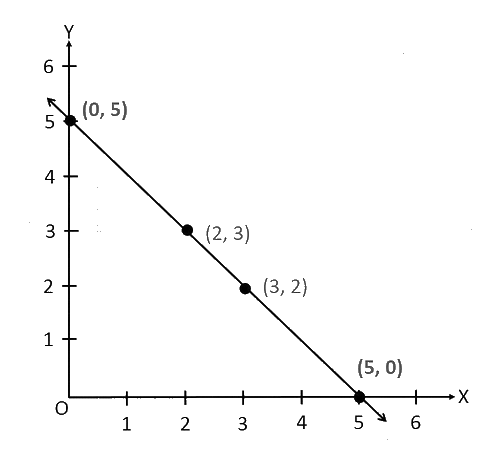# Ex.15.2 Q2 Introduction to Graphs Solutions - NCERT Maths Class 8

Go back to  'Ex.15.2'

## Question

Draw the line passing through ($$2, 3$$) and ($$3, 2$$). Find the coordinates of the points at which this line meets the $$x$$-axis and $$y$$-axis.

Video Solution
Introduction To Graphs
Ex 15.2 | Question 2

## Text Solution

Steps:From the graph, it can be observed that the line joining the points ($$2, 3$$) and ($$3, 2$$) meets the x-axis at the point ($$5, 0$$) and the y-axis at the point ($$0, 5$$).

Learn from the best math teachers and top your exams

• Live one on one classroom and doubt clearing
• Practice worksheets in and after class for conceptual clarity
• Personalized curriculum to keep up with school